Next: 2. Fields Up: 1. Notation, Undefined Concepts Previous: 1.5 Functions   Index

# 1.6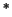Russell's Paradox

There are some logical paradoxes connected with the theory of sets. The book The Foundations of Mathematics by Evert Beth discusses 17 different paradoxes[9, pp. 481-492]. Here I discuss just one of these which was published by Bertrand Russell in 1903[43, ¶78,¶¶ 100-106].

Let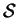be the set of all sets, let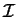be the set of all infinite sets, and let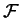be the set of all finite sets. Then we have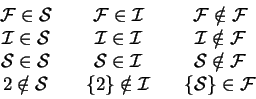Here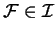since there are infinitely many finite sets.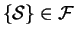since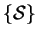contains just one element, which is the set of all sets. Alsosinceis not a set. Next, let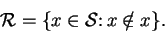Then for allwe have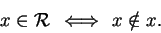(1.59)

Thus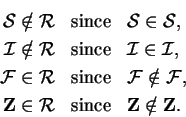We now ask whether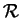is in. According to (1.59),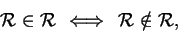i.e.,is inif and only if it isn't!

I believe that this paradox has never been satisfactorily explained. A large branch of mathematics (axiomatic set theory) has been developed to get rid of the paradox, but the axiomatic approaches seem to build a fence covered with  keep out" signs around the paradox rather than explaining it. Observe that the discussion of Russell's paradox does not involve any complicated argument: it lies right on the surface of set theory, and it might cause one to wonder what other paradoxes are lurking in a mathematics based on set theory.